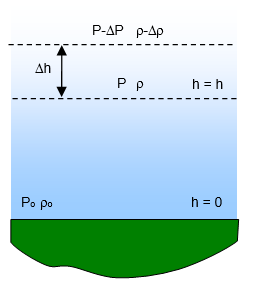# Pressure in compressible fluidsIn working out the pressure at a given depth in water we assume that the water is incompressible – that is its density is constant with depth. This is very nearly accurate. However this would not be true of a fluid such as air. In the atmosphere the pressure increases with depth but the density of the air also varies from 1.2 kgm-3 at sea level to virtually zero at the edge of space.

Let the change in pressure due to a small change of height Δh be Δp

Take the density at a height h to be ρ and the density at the height h+Δh to be ρ-Δρ.

If the temperature (T) of the air is constant ρ is directly proportional to the pressure (p) and so:

p/po = ρ/ρo where po and ρo are the pressure and density of air at sea level.

Therefore Δp/Δh = - rg = -gρo[p/po]

When this is integrated we have:

Pressure (p) at a height h = poe-g[ρo/po]h

Density (ρ) at a height h = ρoe-g[ρo/po]h

Substituting for the accepted values of g, po and ro we have:

Pressure (p) at a height h = poe-0.116h

(N.B – the height h should be given in kilometres here)

Example problem
1. Calculate the pressure of the air at a height of 10 000 m (10 km) (the height at which many intercontinental jets fly). Take the pressure at sea level to be 105 Pa.

Pressure (p) = 105e-0.116h
Pressure = 105xe-1.16 = 3.13x104 Pa or about a third of that at sea level.

2. Calculate the distance below the surface of the Earth at which a piece of iron (density 7870kgm-3) will float in air if the density of air at sea level is 1.3 kgm-3.

7870 = 1.3e-0.116h
Therefore 6053 = e-0.116h giving h = 75 km!

The temperature of the atmosphere also decreases with height.
The standard variation being a drop of 6 oC for every kilometre above the ground up to 11 km. After that it remains constant at around – 55 oC.

A VERSION IN WORD IS AVAILABLE ON THE SCHOOLPHYSICS USB